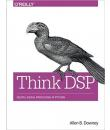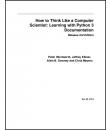Меню

# Doing Math with Python. A. Saha

## Описание

This book’s goal is to bring together three topics near to my heart—programming, math, and science. What does that mean exactly? Within these pages, we’ll programmatically explore high school–level topics, like manipulating units of measurement; examining projectile motion; calculating mean, median, and mode; determining linear correlation; solving algebraic equations; describing the motion of a simple pendulum; simulating dice games; creating geometric shapes; and finding the limits, derivatives, and integrals of functions. These are familiar topics for many, but instead of using pen and paper, we’ll use our computer to explore them.
We’ll write programs that will take numbers and formulas as input, do the tedious calculations needed, and then spit out the solution or draw a graph. Some of these programs are powerful calculators for solving math problems. They find the solutions to equations, calculate the correlation between sets of data, and determine the maximum value of a function, among other tasks. In other programs, we’ll simulate real-life events, such as projectile motion, a coin toss, or a die roll. Using programs to simulate such events gives us an easy way to analyze and learn more about them.

### Схожие книгиPython
en

#### Django Project Blueprints. Asad Jibran AhmedPython
en

#### Think DSP Digital Signal Processing in Python. Allen B. DowneyPython
en

#### Black hat Python. Justin SeitzPython
en